# Problem 53109. Easy Sequences 52: Non-squarable Rectangles

Any integer-sided rectangle can be cut into unit rectangles (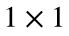) and rearranged into sets of smaller rectangles. For example the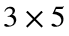rectangle can be broken as follows: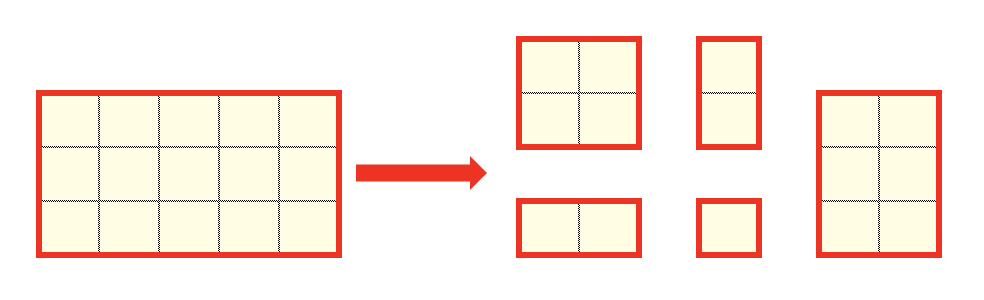We call an integer rectangle as "squarable" if it can be can be broken (i.e. cut into unit rectangles and rearranged) into any number of non-unit squares of equal sizes. For example the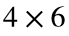rectangle can be broken into six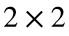squares. Therefore, therectangle is squarable.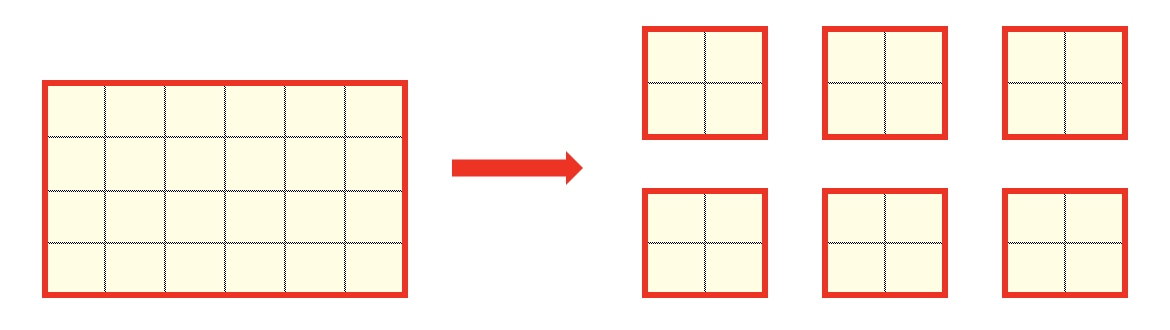Integer rectangles that are not squarable are called "non-squarable". Therectangle, shown in the first example above, is a non-squarable rectangle. The complete set of non-squarable rectangles with area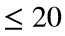square units are as follows: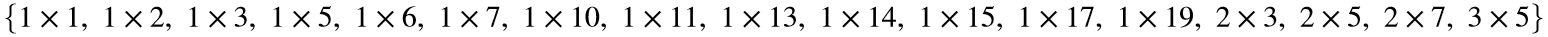Create a program that calculates the total area of all non-squarable integer rectangles whose areas are less than or equal to a given area limit A.
For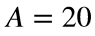, the program should output: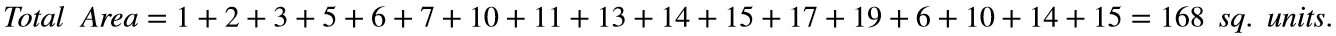NOTE: Reflections and rotations are not significant and should be counted only once.

### Solution Stats

75.0% Correct | 25.0% Incorrect
Last Solution submitted on Jun 30, 2023

### Community Treasure Hunt

Find the treasures in MATLAB Central and discover how the community can help you!

Start Hunting!